# How to Calculate and Solve for Mass, Angular Velocity, Radius and Centrifugal Force of a Body | The Calculator Encyclopedia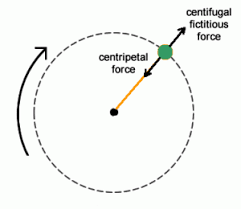The image above represents the centrifugal force.

To compute for the centrifugal force, three essential parameters are needed and these parameters are Mass of the body (m), Angular Velocity of the body (w) and Radius (r).

The formula for calculating the centrifugal force:

F = mω²r

Where:
F = Centrifugal Force
m = mass of the body
ω = angular velocity

Let’s solve an example;
Find the centrifugal force with mass of the body as 12, angular velocity as 32 and a radius of 8.

This implies that;
m = mass of the body = 12
ω = angular velocity = 32

F = mω²r
F = 12 x 32² x 8
F = 12 x 1024 x 8
F = 98304

Therefore, the centrifugal force is 98304 N.

Calculating the Mass of the body (m) when the Centrifugal Force, Angular Velocity and Radius is Given.

m = F / w2r

Where;
m = mass of the body
F = Centrifugal Force
ω = angular velocity

Let’s solve an example;
Find the mass of a body when centrifugal force is 140 with an angular velocity of 24 and a radius of 10.

This implies that;
F = Centrifugal Force = 140
ω = angular velocity = 24

m = F / w2r
m = 140 / 24210
m = 140 / 576 x 10
m = 140 / 5760
m = 0.024

Therefore, the mass of the body is 0.024 kg.

Calculating the Angular Velocity (w) when the Centrifugal Force, Mass of the body (m) and Radius is Given.

w = √F / mr

Where;
ω = angular velocity
m = mass of the body
F = Centrifugal Force

Let’s solve an example;
Find the angular velocity when centrifugal force is 220 with a mass of the body of 14 and a radius of 6.

This implies that;
F = Centrifugal Force = 220
m = mass of the body = 14

w = √F / mr
w = √220 / 14 x 6
w = √220 / 84
w = √2.619
w = 1.618

Therefore, the angular velocity is 1.618.

Calculating the Radius (r) when the Centrifugal Force, Mass of the body (m) and Angular Velocity is Given.

r = F / mw2

Where;
F = Centrifugal Force
ω = angular velocity
m = mass of the body

Let’s solve an example;
Find the radius with a centrifugal force of 280, mass of the body is 16 and an angular velocity of 22.

This implies that;
F = Centrifugal Force = 280
ω = angular velocity = 22
m = mass of the body = 16

r = F / mw2
r = 280 / 16 x 222
r = 280 / 16 x 44
r = 280 / 704
r = 0.397

Nickzom Calculator – The Calculator Encyclopedia is capable of calculating the centrifugal force.

To get the answer and workings of the centrifugal force using the Nickzom Calculator – The Calculator Encyclopedia. First, you need to obtain the app.

You can get this app via any of these means:

To get access to the professional version via web, you need to register and subscribe for NGN 1,500 per annum to have utter access to all functionalities.
You can also try the demo version via https://www.nickzom.org/calculator

Once, you have obtained the calculator encyclopedia app, proceed to the Calculator Map, then click on Mechanics under EngineeringNow, Click on Motion of Circular Path under Mechanics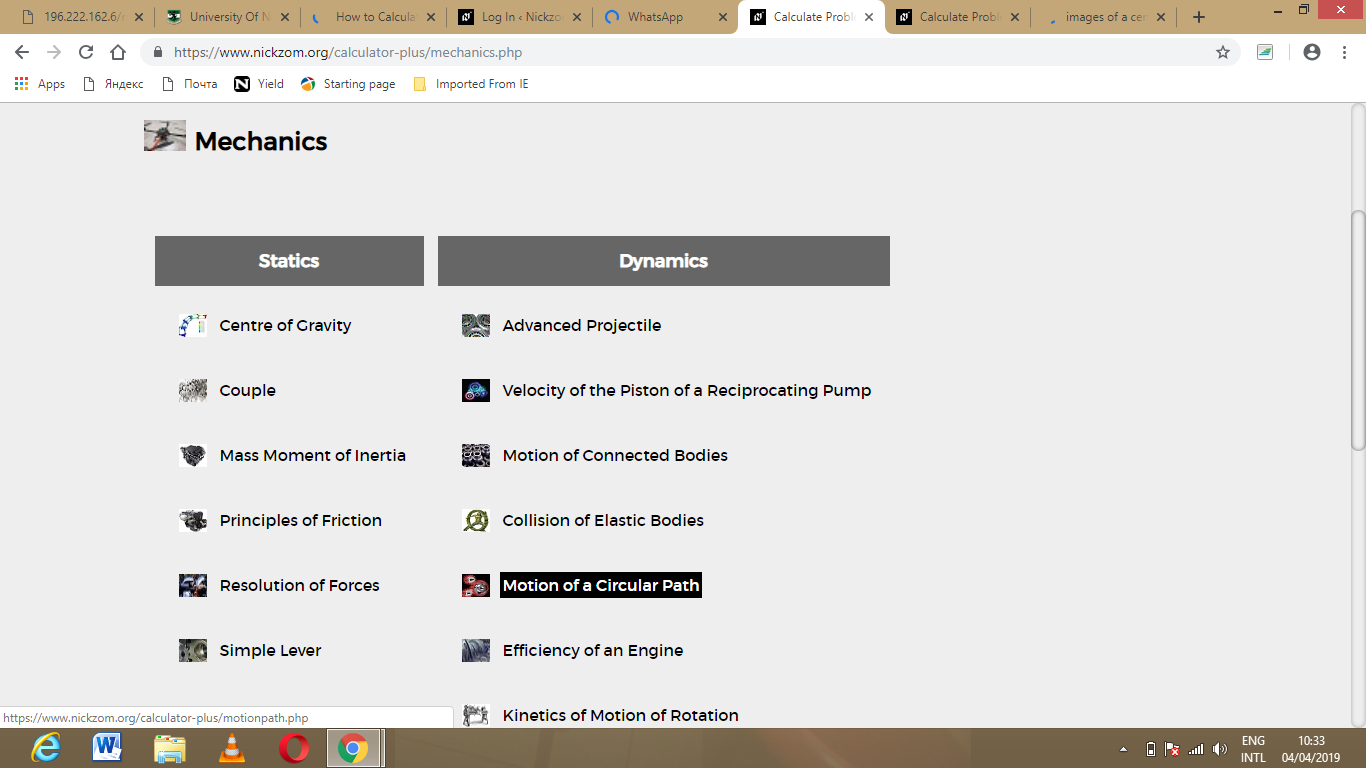Click on Centrifugal Force under Motion of Circular PathThe screenshot below displays the page or activity to enter your values, to get the answer for the centrifugal force according to the respective parameters which are the mass of the body (m), angular velocity (v) and radius (r).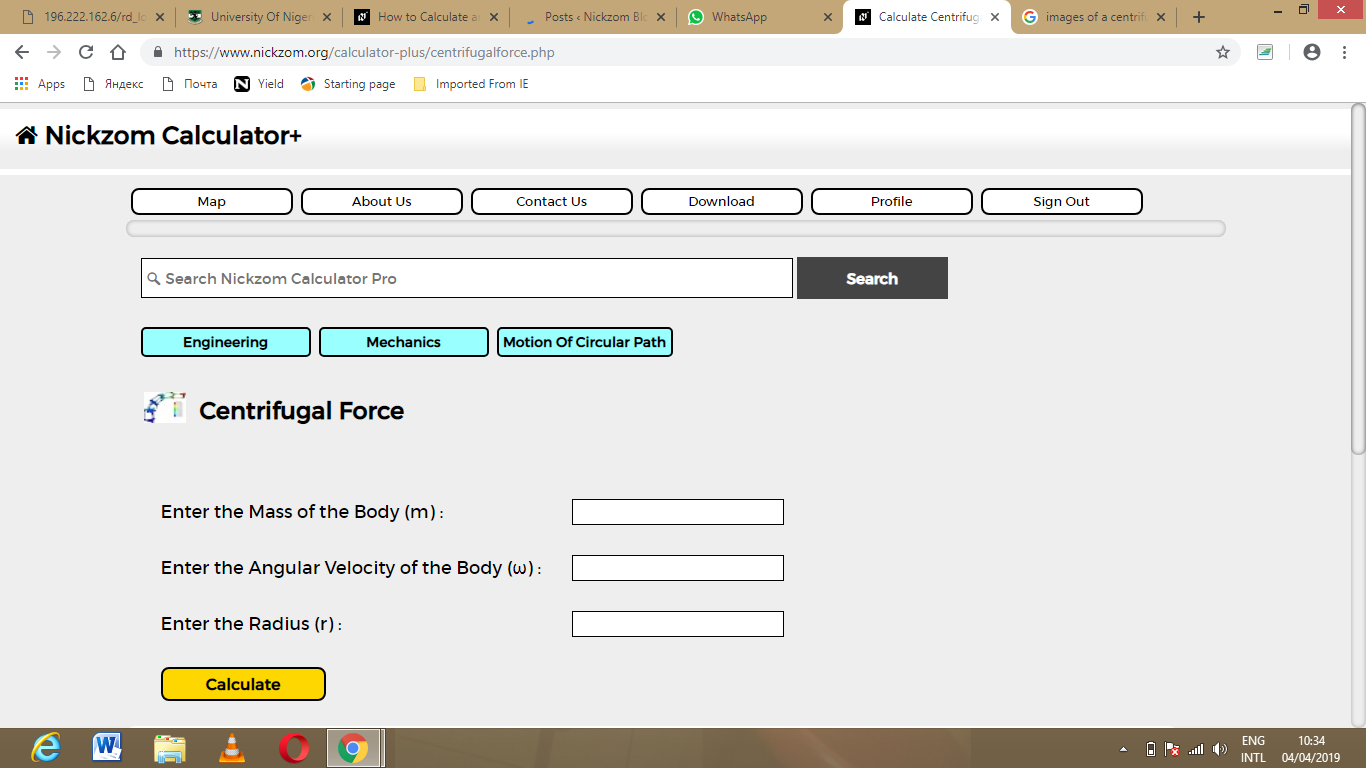Now, enter the values appropriately and accordingly for the parameters as required by the mass of the body (m) is 12, angular velocity (v) is 32 and radius (r) is 8.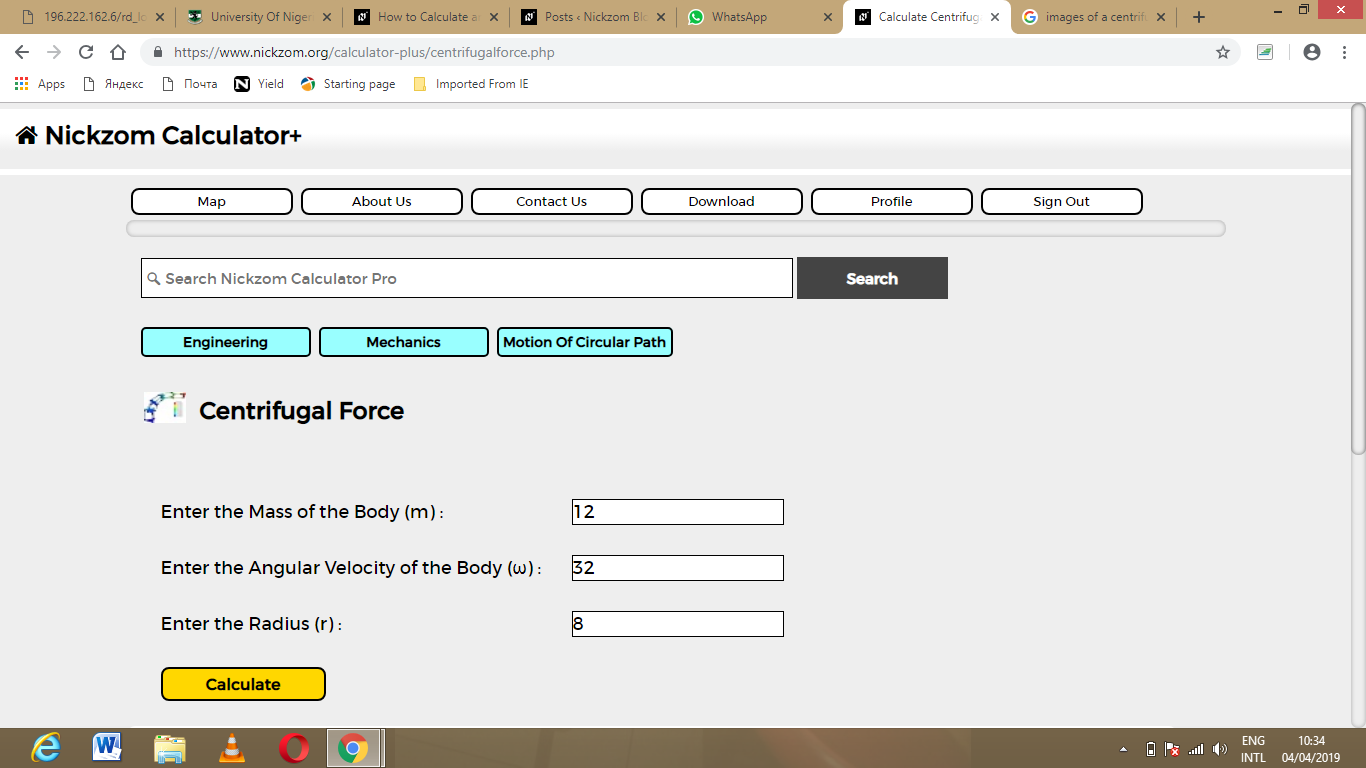Finally, Click on Calculate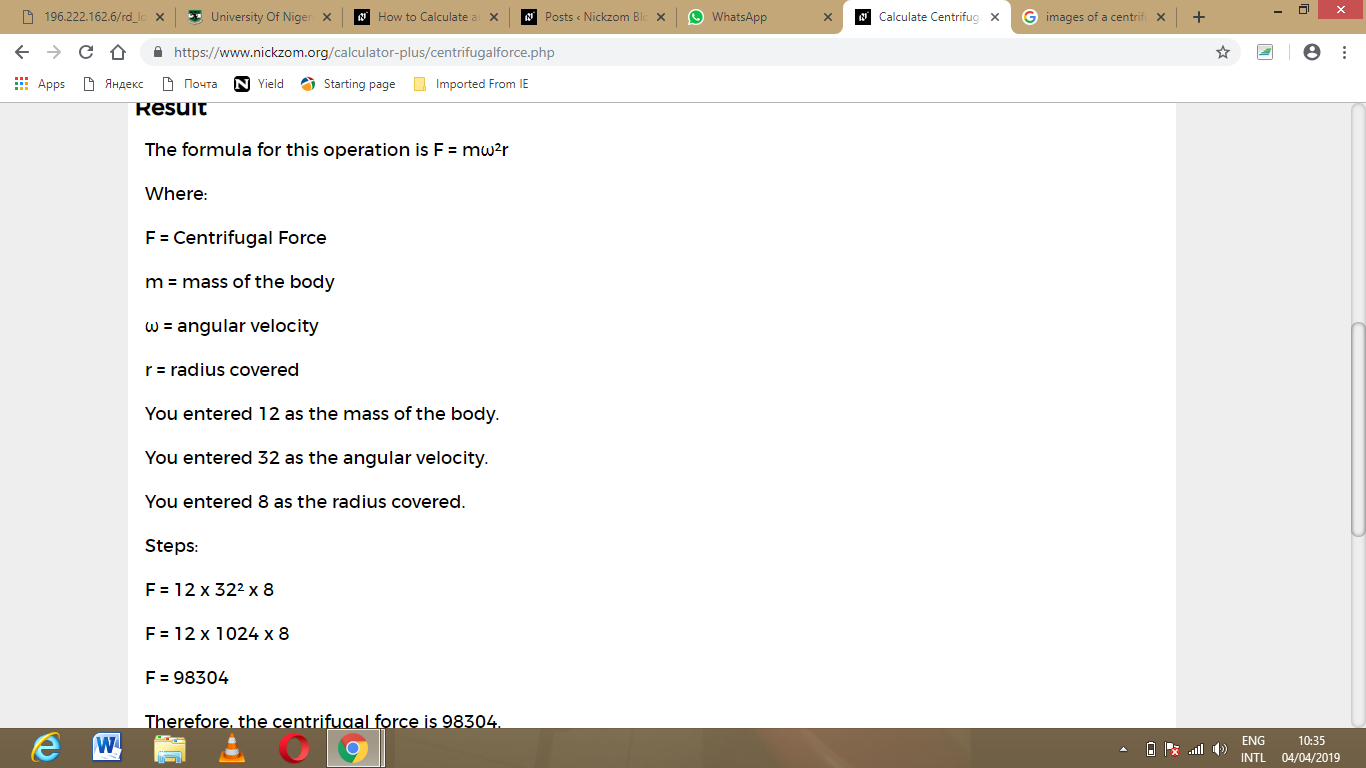As you can see from the screenshot above, Nickzom Calculator – The Calculator Encyclopedia solves for the centrifugal force and presents the formula, workings and steps too.